# RD Sharma Class 10 Solutions Chapter 15 Statistics Ex 15.2

In this chapter, we provide RD Sharma Solutions for Class 10 Chapter 15 Statistics Ex 15.2 for English medium students, Which will very helpful for every student in their exams. Students can download the latest RD Sharma Solutions for Class 10 Chapter 15 Statistics Ex 15.2 pdf, free RD Sharma Solutions for Class 10 Chapter 15 Statistics Ex 15.2 book pdf download. Now you will get step by step solution to each question.

## RD Sharma Class 10 Solutions Chapter 15 Statistics Ex 15.2

Question 1.
The. number of telephone calls received at an exchange per interval for 250 successive one-minute intervals are given in the following frequency table:Compute the mean number of calls per interval.
Solution:
Let assumed mean (A) = 4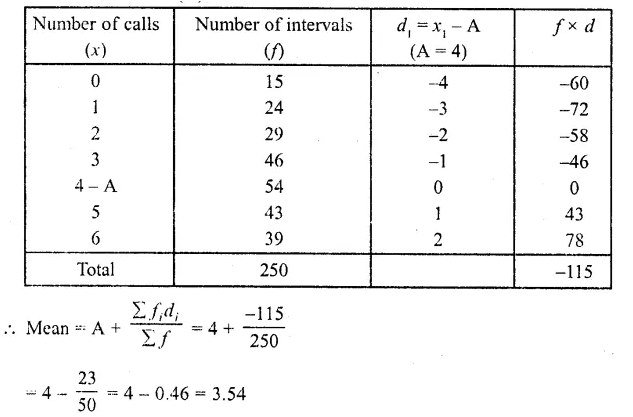Hence mean number of calls per interval = 3.54

Question 2.
Five coins were simultaneously tossed 1000 times, and at each toss the number of heads was observed. The number of tosses during which 0, 1, 2, 3, 4, and 5 heads were obtained are shown in the table below. Find the mean number of heads per tossSolution:
Let assumed means (A) = 3Hence mean number of tosses per head = 2.47

Question 3.
The following table gives the number of branches and number of plants in the garden of a school.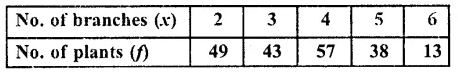Calculate the average number of branches per plant.
Solution:
Let assumed mean (A) = 4∴Mean number of branches per plant = 3.62

Question 4.
The following table gives the number of children of 150 families in a villageFind the average number of children per family.
Solution:
Let assumed mean (A) = 3= 3 – 0.65 = 2.35
Hence mean number of children per family = 2.35

Question 5.
The marks obtained out of 50, by 102 students in a Physics test are given in the frequency table below:Find the average number of marks.
Solution: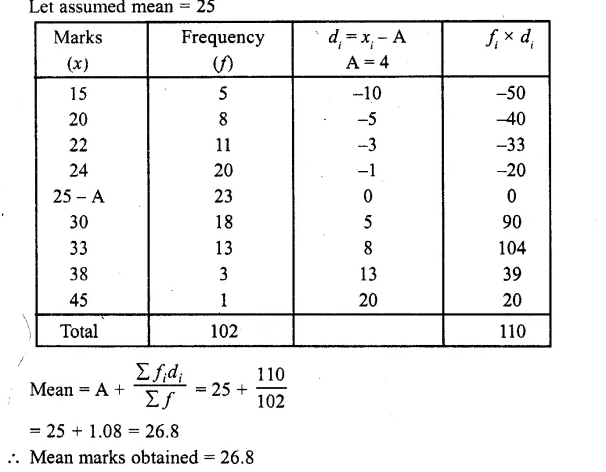Question 6.
The number of students absent in a class were recorded every day for 120 days and theSolution:
Let assumed mean = 3= 3 + 0.525 = 3.525 = 3.53 (approx)

Question 7.
In the first proof reading of a book containing 300 pages the following distribution of misprints was obtained: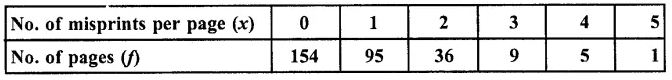Find the average number of misprints per page.
Solution:
Let assumed mean (A) = 2= 2 – 127 = 0.73
∴ Average of number of misprints per page = 0.73

Question 8.
The following distribution gives the number of accidents met by 160 workers in a factory during a month.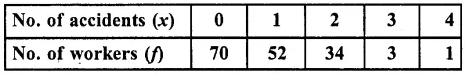Find the average number of accidents per worker.
Solution:
Let assumed mean = 2= 2 – 1.168 = 2 – 1.17 = 0.83 (approx)
∴ Average number of accidents per worker = 0.83

Question 9.
Find the mean from the following frequency distribution of marks at a test in statistics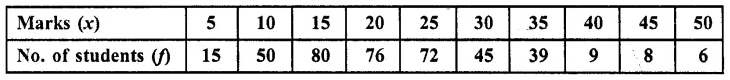Solution:
Let assumed mean = 25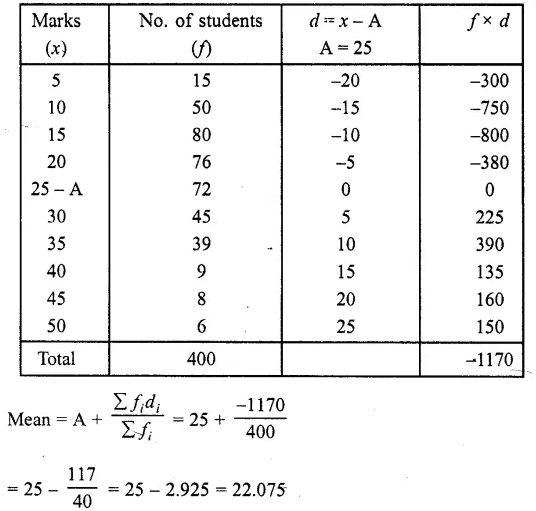∴Average of marked obtained per student = 22.075

All Chapter RD Sharma Solutions For Class10 Maths

—————————————————————————–

All Subject NCERT Exemplar Problems Solutions For Class10

All Subject NCERT Solutions For Class 10

*************************************************

I think you got complete solutions for this chapter. If You have any queries regarding this chapter, please comment on the below section our subject teacher will answer you. We tried our best to give complete solutions so you got good marks in your exam.

If these solutions have helped you, you can also share rdsharmasolutions.in to your friends.